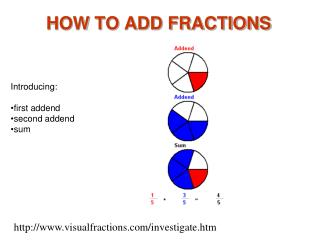DownloadDownload PresentationDownload Presentation- - - - - - - - - - - - - - - - - - - - - - - - - - - E N D - - - - - - - - - - - - - - - - - - - - - - - - - - -
##### Presentation Transcript

2. Add Fractions 1 This picture shows an addition example with two addends and a sum. The first addend1/5 is combined with the second addend3/5 to give the sum4/5. Notice how the sum4/5 is combines the red 1/5 with the blue 3/5.

3. Add Fractions 2 1/5 and 3/5 are like fractions because the denominators are the same. When the addend denominators are the same, add the numerators to get the numerator of the sum.

4. Add Fractions 3 The sum12/8 is written as a mixed number 1 4/8 and is then written in lowest terms. The numerals 12/8 , 1 4/8 and 1 1/2 are all correct names for the sum of 5/8 and 7/8.

5. Add Fractions 4 Here, mixed numbers are added. The whole number 1 in 1 3/5 is added to the whole number 2 in 2 1/5 for a whole number 3 in the sum. The fractions 3/5 and 1/5 are added for 4/5 in the sum.

6. Add Fractions 5 The same example 1 3/5 plus 2 1/5 is shown with number lines. Add the whole numbers and then the fractions: 1 3/5 + 2 1/5 = (1+2)+ (3/5 + 1/5) = 3 4/5.

7. Add Fractions 6 This example shows the sum 3 5/5 written as 4. Since the fraction 5/5 is equal to 1, 3 5/5 is equal to 3 + 1 for a sum of 4

8. Add Fractions 7 This example shows the sum 3 7/5 written as 4 2/5. The 7/5 part of the sum can be renamed as 1 2/5 . The 1 in 1 2/5 is added to the whole number 3 for the 4 in 4 2/5: 1 3/5 + 2 4/5 = 3 7/5 = 3 + 1 2/5 = 4 2/5.

9. Add Fractions 8 The addends 2/3 and 3/5 are unlike fractions. Each addend is written with the common denominator 15 before the numerators are added, giving a sum of 19/15 or 1 4/15.

10. Add Fractions 9 The unlike fractions 21/4 and 12/3 are renamed as like fractions 23/12 and 18/12. Then the whole numbers and numerators are added for a sum of 33/12.

11. Add Fractions 10 Write each addend with a common denominator 12. Because 17/12 can be written as 15/12, we can write the sum 4 17/12 as 5 5/12.